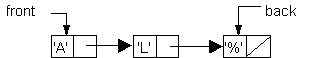Pointer Implementation of Queues

 Use this diagram to answerthe following questions.1. How many nodes does this queue have?
A. 1
B. 2
C. 3
D. 4

2. What steps are necessary when deleting a node from this queue?
A. Set rear to point to the node immediately in front of the last node in the queue and dispose of the last node.
B. Set the last node to point to rear and dispose of the last node.
C. Set the next to the last node to point to the last node and dispose of the last node.
D. Set front equal to the node immediately following the first node and dispose of the first node.

3. What steps are necessary when adding a node to this queue?
A. Set the pointer field of the rear of the list to the new node and set the rear pointer to point to the new node.
B. Set the new node's pointer field equal to the value of the rear pointer and set rear equal to the address of the node immediately in front of the new node.
C. Set the new node's pointer field equal to front and set front to point to the new node.
D. Set the new node's pointer field equal to the value of the front pointer and set the front pointer to point to the rear of the list.

4. When traversing this queue, how can it be determined if the end has been reached?
A. when rear is equal to `nil`
B. when the data field of the current node is equal to zero
C. when the link field of the node currently being accessed is equal to `NULL`
D. when a run-time error occurs

5. Given the following declarations, identify and correct the errors in the segment.
```struct node
{
int jobNumber;
};
struct Qnode
{
node* front;
node* rear;
};
Qnode Q;

void dequeue (Qnode &Q, int &nextJob)
// Removes first job number from queue
{
node* temp;
if ( Q.isEmpty( ) )
cout << "Queue is empty." << endl;
else
{
nextJob = Q.front -> jobNumber;
temp = Q.rear